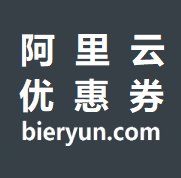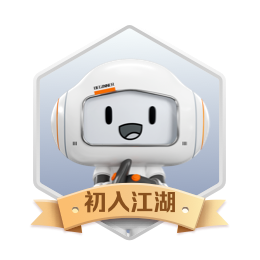# PHP之从反向删除单链表元素的问题谈起

+关注继续查看

PHP之从反向删除单链表元素的问题谈起

PHP7.0版本除了对象，资源之外，其余数据类型均已实现写时复制

<?php

$obj1 = new stdClass();$obj1->val = 3;
$obj1->next = null;$obj2 = $obj1;$obj2->next = array();

$obj2 = null; var_dump($obj1);

• public $next = null; • function __construct($val) { $this->val =$val; }
• }
*/

class Solution {

/**
* @param ListNode $head * @param Integer$n
* @return ListNode
*/
function removeNthFromEnd($head,$n) {
$length = getLength($head);
$from_start_num =$length - $n; // if ($n == 1 && $length == 1) { // return null; // } // if ($from_start_num == 0 && $n ===$length) {
//     return $head->next; // }$return_node_list = deleteLinkItem($head,$from_start_num);
return $return_node_list; } } /** • @param$head
• @param int $index • @return null */ function deleteLinkItem($head, $index = 1) { if (is_null($head)) {
return null;
} else {
if ($index == 0) { return$head->next;
}
$i = 1;$current = $head; while($current->next != null) {
if ($i ==$index) {
$tmp =$current->next;
break;
}
$i++;$current = $current->next; }$current->next = $tmp->next;   return$head;
}


}

/**

• @param $head • @param$node
• @param int $index • @return mixed */ function getLength($head) {

if (empty($head)) { return 0; }$i = 1;
while($head->next != null) {$i++;
$head =$head->next;
}

return $i; } 唯一要注意的是，由于deleteLinkItem的$index是从1开始算的，和脚标起始0不同，假如是要删除0下标（也就链表是第一个元素被删除），那么直接返回头部节点的next即可，如代码中的这段：

if ($index == 0) { return$head->next;

}PHP执行批量mysql语句
1207 0848 0apache+mysql+php编译参数
767 0[PHP] PHP+MYSQL留言板制作
2001 0PHP到MySQL数据查询过程概述
1778 01307 01647 01652 02231 0centos下 Apache、php、mysql默认安装路径
apache: 如果采用RPM包安装，安装路径应在 /etc/httpd目录下 apache配置文件:/etc/httpd/conf/httpd.conf Apache模块路径：/usr/sbin/apachectl web目录:/var/www/html 如果采用源代码安装，一般默认安装在/usr/local/apache2目录下 php: 如果采用RPM包安装，安装路
3018 0+关注

569

4